## Algebra

Showing posts with label check solution. Show all posts
Showing posts with label check solution. Show all posts

Now that we have learned how to work with radical expressions, we next move on to solving.

Use caution when solving radical equations because the following steps may lead to extraneous solutions, solutions that do not solve the original equation.

Solve:Step 2: Square both sides of the equation.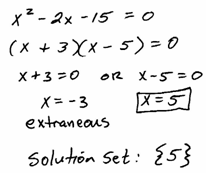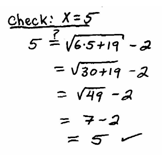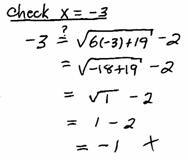Whenever you raise both sides of an equation to an even power, you introduce the possibility of extraneous solutions so the check is essential here.

Solve.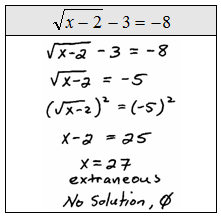The index determines the power to which we raise both sides.  For example, if we have a cube root we will raise both sides to the 3rd power. The property that we are using isfor integers n > 1 and positive real numbers x. After eliminating the radical, we will most likely be left with either a linear or a quadratic equation to solve.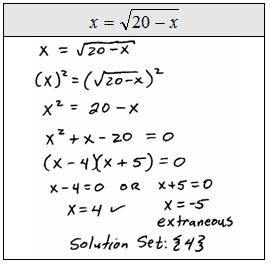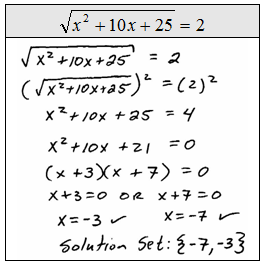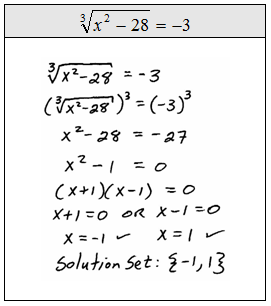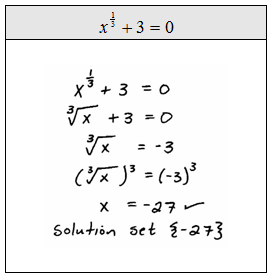The check mark indicates that we have actually checked that the value is a solution to the equation, do not dismiss this step, it is essential.Some radical equations have more than one radical expression.  These require us to isolate each remaining radical expression and raise both sides to the nth power until they are all eliminated.  Be patient with these, go slow and avoid short cuts.
Solve: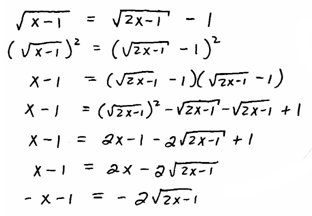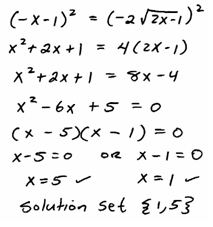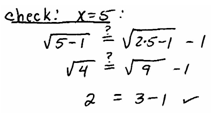Solve: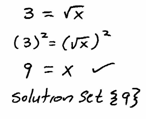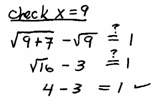Solve: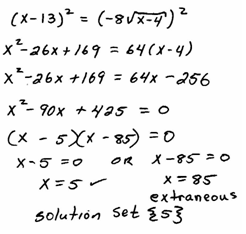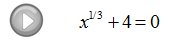### Solving Linear Equations: Part I

One method for solving a linear equation that looks like 2x + 3 = 13 is to simply guess and check.  What value can I substitute in for x so that the result will be 13?  After some thought it should be easy to see that x must equal 5 because 2 * 5 + 3 = 10 + 3 = 13.  However, guessing at solutions to linear equations becomes very difficult when the numbers are not so nice.  When solving, the idea is to use the properties of equality to isolate the variable.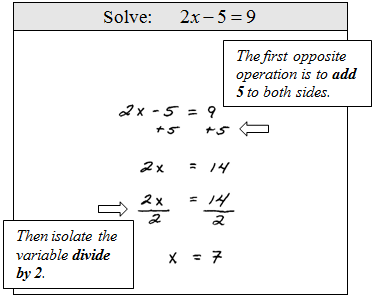Tip: We can always check by substituting our solution into the original equation to see if we obtain a true statement.  If you have time on an exam you should always check.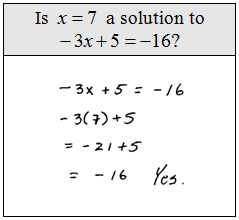Solve for x.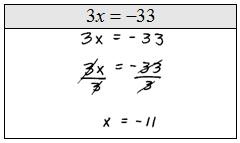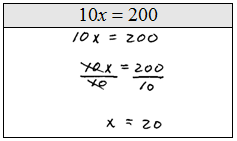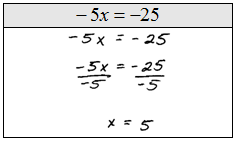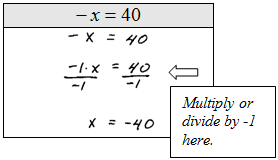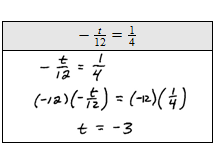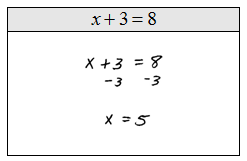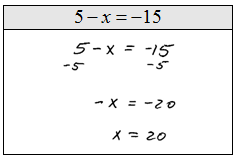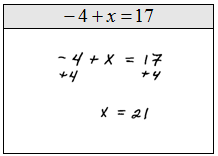Word Problem: Maria scored 7 more points than Jose on the first mathematics exam.  If Maria's total score was 95 what was Jose's total. (Set up an algebraic equation and solve it)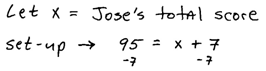Tip: You can save a step if you multiply both sides of the equation by the reciprocal of the coefficient.When solving, apply the addition or subtraction property of equality first. Next apply the multiplication or division property of equality as needed to isolate the variable.

Solving General Two Step Equations
Steps for solving general linear equations of the form $ax + b = c$.
$$2x + 3 = 13$$
Add $-3$ on both sides of the equation.
$$2x = 10$$
Divide both sides by $2$.
$$x = 5$$
Check: $2(5) + 3 = 10 + 3 = 13$ ✔

Solve for x.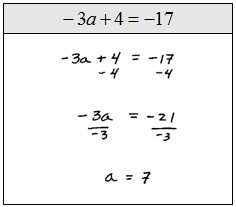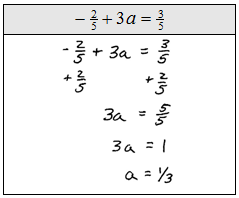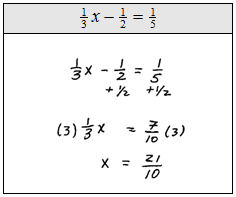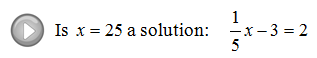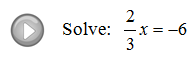---